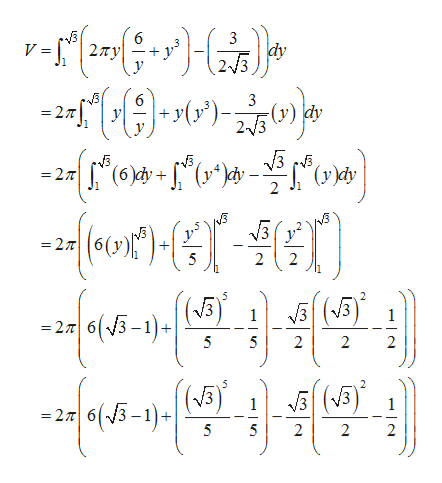What is the volume of this equation using the shell method?2(pi)y((6/y+y^3)-(3/2(sqrt3))dy from the integral [1,sqrt3]

Question

What is the volume of this equation using the shell method?

2(pi)y((6/y+y^3)-(3/2(sqrt3))dy from the integral [1,sqrt3]

Step 1

The volume for the given interval over the interv...help_outlineImage Transcriptionclose6 27Ty 3 dy 2/3 V = 3 (r); = 27 23) dy 1 (6)dy (y^^4y 27 2 =277 2 2 5 2 V3 33 1 1 |s(5-1) =27 6 5 5 2 2 2 2 (3 |(5-1)+ V3 1 2 6 5 5 2 2 2 fullscreen

Want to see the full answer?

See Solution

Want to see this answer and more?

Our solutions are written by experts, many with advanced degrees, and available 24/7

See Solution
Tagged in

Integration